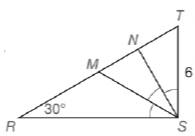Chapter 5.6, Problem 40EElementary Geometry For College St...

7th Edition
Alexander + 2 others
ISBN: 9781337614085

Solutions

Chapter
SectionElementary Geometry For College St...

7th Edition
Alexander + 2 others
ISBN: 9781337614085
Textbook Problem

Given: Δ R S T with right ∠ R S T ; m ∠ R = 30 ∘ and S T = 6 ; ∠ R S T is trisected by S M → and S N →Find: T N , N M , and M R

To determine

To find:

TN,NM,MR.

Explanation

Given:

In a ΔRST with right RST, mR=30,ST=6 and RST is trisected by SM,SN as shown below

Theorem used:

Angle bisector theorem:

If a ray bisects one angle of a triangle, then it divides the opposite side into segments whose lengths are proportional to the lengths of the two sides that form the bisected angle.

Calculation:

Consider a ΔRST with right RST.

Since the RST is trisected, which is a right angle, we get mTSN=30,mNSM=30,mMSR=30.

Given that mR=30

mMSR=mR

Therefore triangle RMS is an isosceles triangle with MR=MS.

From the figure,

mTSM=mTSN+mNSM=30+30=60

mT=90mTRS=9030=60

Still sussing out bartleby?

Check out a sample textbook solution.

See a sample solution

The Solution to Your Study Problems

Bartleby provides explanations to thousands of textbook problems written by our experts, many with advanced degrees!

Get Started

In Exercises 73-80, find the indicated limits, if they exist. 77. limxx4+1x31

Applied Calculus for the Managerial, Life, and Social Sciences: A Brief Approach

Describe the set of points (x,y) such that x2+y2=0.

Finite Mathematics and Applied Calculus (MindTap Course List)

True or False: If and , then .

Study Guide for Stewart's Multivariable Calculus, 8th

If A1 and A2 are the areas at the right then abf(x)dx= a) A1 A2 b) A1 + A2 c) A1 + A2 d) A1A2

Study Guide for Stewart's Single Variable Calculus: Early Transcendentals, 8th